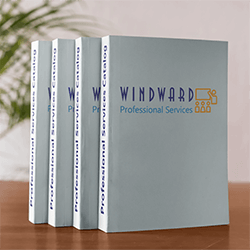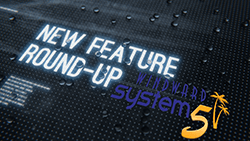#Windward Software Wiki

## Get the Most Out of Your ERPOur catalog of services has topic-based, role-based, or technical plans that can help you maximize your software investment.

### What's New in Windward System Fivesato_cg408dt_labels_samples

# Explanation

### The SATO printers don't require code based labels. They will work with the Windward Label Designer.

However, Code Based Labels will give you more control over how barcodes are printed, and code based labels will print faster than labels created in the System Five Label Designer.

The code used for the CG408DT does vary slightly from the SATO CG408DT-RS. So you can not use the CG408DT-RS codes. It will throw the printer into an error, or not print a label at all. You will need to refer to the “SATO Programming Reference.pdf” for this printer. This printer's codes are closer to the LM408.

The '8' in printer model 'CG408' or 'LM408' refers to a printer that prints 8 dots per mm, or 203 dots per inch. Therefore a measurement of 50 dots rounds out to be 1/4 inch, etc.

# Printing Barcode vs. Barcode Numbers

There has also been some confusion regarding Barcode text lines and actual Barcodes. The System Five field <barcode> is the same, but the information to print the barcode number, or the barcode are completely different. For printing just the barcode number on a label, you will need to tell the printer what font you want to print as well as the size, orientation, and position on the label.

But for a barcode, you need to supply different information, such as the barcode type (3of9, 128, or UPC), the height and width of the barcode, and the position on the label.

# Label Sample 1

#### 2" x 4" Label

```<STX>
<ESC>A<ESC>A108120406<ESC>Z
<ESC>A
<ESC>%1<ESC>H10<ESC>V800<ESC>\$A,050,060,0<ESC>\$=<COMPANY.NAME,-1>
<ESC>%1<ESC>H110<ESC>V800<ESC>\$A,040,050,0<ESC>\$=<DESCRIPTION,-1>
<ESC>%1<ESC>H190<ESC>V800<ESC>\$A,040,050,0<ESC>\$=<PART,-1>
<ESC>%1<ESC>H250<ESC>V800<ESC>BG02100<Barcode,-1>
<ESC>%1<ESC>H350<ESC>V800<ESC>\$A,025,030,0<ESC>\$=<Barcode,-1>
<ESC>%1<ESC>H235<ESC>V300<ESC>\$A,025,030,0<ESC>\$=PRICE
<ESC>%1<ESC>H260<ESC>V300<ESC>\$A,090,100,0<ESC>\$=<PRICE.0,-1>
<ESC>Q1
<ESC>Z
<ETX>
```

# Explanation of Lines

##### <ESC>A<ESC>A108120406<ESC>Z
• (label size in dots. A1aaaabbbb - aaaa is the label height and bbbb is the label width. In this case 0812 x 0406. There are 203 dots per inch (8 dots per mm))
##### <ESC>A
• (start of label)
##### <ESC>%1<ESC>H10<ESC>V800<ESC>\$A,050,060,0<ESC>\$=<COMPANY.NAME,-1>
• (%1 is the rotation of the information 0=normal, 1=90 degrees, 2=180 degrees(upside down), and 3=270 degrees)
• (Hxxx tells the printer what horizontal position to start printing at)
• (Vxxx tells the printer what vertical position to start printing at)
• (<ESC>\$ is a vector font type - A is Helvetica Bold (proportional spacing))
• (50 is the font width in dots @ 203 dots per inch (8 dots per mm))
• (60 is the font height in dots @ 203 dots per inch (8 dots per mm))
• (0 is the font variation - 0: Standard, 1: Standard open (outlined), 2: Gray (mesh) pattern 1, 3: Gray (mesh) pattern 2, 4: Gray (mesh) pattern 3, 5: Standard open, shadow 1, 6: Standard open, shadow 2, 7: Standard mirror image, 8: Italic, 9 Italic open, shadow
• (<ESC>\$=<COMPANY.NAME,-1> This is the System Five field used to display the Company Name)
##### <ESC>%1<ESC>H110<ESC>V800<ESC>\$A,040,050,0<ESC>\$=<DESCRIPTION,-1>
• (%1 is the rotation of the information 0=normal, 1=90 degrees, 2=180 degrees(upside down), and 3=270 degrees)
• (Hxxx tells the printer what horizontal position to start printing at)
• (Vxxx tells the printer what vertical position to start printing at)
• (<ESC>\$ is a vector font type - A is Helvetica Bold (proportional spacing))
• (40 is the font width in dots @ 203 dots per inch (8 dots per mm))
• (50 is the font height in dots @ 203 dots per inch (8 dots per mm))
• (0 is the font variation - 0: Standard, 1: Standard open (outlined), 2: Gray (mesh) pattern 1, 3: Gray (mesh) pattern 2, 4: Gray (mesh) pattern 3, 5: Standard open, shadow 1, 6: Standard open, shadow 2, 7: Standard mirror image, 8: Italic, 9 Italic open, shadow
• (<ESC>\$=<DESCRIPTION,-1> This is the System Five field used to display the description. The -1 means to print the entire description If you need to limit the number of characters, then just delete the - and enter the number of characters you want to print.)
##### <ESC>%1<ESC>H190<ESC>V800<ESC>\$A,040,050,0<ESC>\$=<PART,-1>
• (%1 is the rotation of the information 0=normal, 1=90 degrees, 2=180 degrees(upside down), and 3=270 degrees)
• (Hxxx tells the printer what horizontal position to start printing at)
• (Vxxx tells the printer what vertical position to start printing at)
• (<ESC>\$ is a vector font type - A is Helvetica Bold (proportional spacing))
• (040 is the font width in dots @ 203 dots per inch (8 dots per mm))
• (050 is the font height in dots @ 203 dots per inch (8 dots per mm))
• (0 is the font variation - 0: Standard, 1: Standard open (outlined), 2: Gray (mesh) pattern 1, 3: Gray (mesh) pattern 2, 4: Gray (mesh) pattern 3, 5: Standard open, shadow 1, 6: Standard open, shadow 2, 7: Standard mirror image, 8: Italic, 9 Italic open, shadow
• (<ESC>\$=<PART,-1> This is the System Five field used to display the Part Number. The -1 means to print the entire description If you need to limit the number of characters, then just delete the - and enter the number of characters you want to print.)
##### <ESC>%1<ESC>H250<ESC>V800<ESC>BG02100<Barcode,-1>
• (%1 is the rotation of the information 0=normal, 1=90 degrees, 2=180 degrees(upside down), and 3=270 degrees)
• (Hxxx tells the printer what horizontal position to start printing at)
• (Vxxx tells the printer what vertical position to start printing at)
• (BG Prints a CODE 128 barcode 01 is the narrow bar width, 100 is the barcode height)
• (<BARCODE,-1> This is the System Five field used to display the barcode. The -1 means to print the entire barcode)
##### <ESC>%1<ESC>H350<ESC>V800<ESC>\$A,025,030,0<ESC>\$=<Barcode,-1>
• (%1 is the rotation of the information 0=normal, 1=90 degrees, 2=180 degrees(upside down), and 3=270 degrees)
• (Hxxx tells the printer what horizontal position to start printing at)
• (Vxxx tells the printer what vertical position to start printing at)
• (<ESC>\$ is a vector font type - A is Helvetica Bold (proportional spacing))
• (025 is the font width in dots @ 203 dots per inch (8 dots per mm))
• (030 is the font height in dots @ 203 dots per inch (8 dots per mm))
• (0 is the font variation - 0: Standard, 1: Standard open (outlined), 2: Gray (mesh) pattern 1, 3: Gray (mesh) pattern 2, 4: Gray (mesh) pattern 3, 5: Standard open, shadow 1, 6: Standard open, shadow 2, 7: Standard mirror image, 8: Italic, 9 Italic open, shadow
• (<ESC>\$=<Barcode,-1> This is the System Five field used to display the Barcode Number. The -1 means to print the entire description If you need to limit the number of characters, then just delete the - and enter the number of characters you want to print.)
##### <ESC>%1<ESC>H235<ESC>V300<ESC>\$A,025,030,0<ESC>\$=PRICE
• (%1 is the rotation of the information 0=normal, 1=90 degrees, 2=180 degrees(upside down), and 3=270 degrees)
• (Hxxx tells the printer what horizontal position to start printing at)
• (Vxxx tells the printer what vertical position to start printing at)
• (<ESC>\$ is a vector font type - A is Helvetica Bold (proportional spacing))
• (025 is the font width in dots @ 203 dots per inch (8 dots per mm))
• (030 is the font height in dots @ 203 dots per inch (8 dots per mm))
• (0 is the font variation - 0: Standard, 1: Standard open (outlined), 2: Gray (mesh) pattern 1, 3: Gray (mesh) pattern 2, 4: Gray (mesh) pattern 3, 5: Standard open, shadow 1, 6: Standard open, shadow 2, 7: Standard mirror image, 8: Italic, 9 Italic open, shadow

* (<ESC>\$=PRICE This is not a System Five field. Notice that there aren't and <> symbols next to the word PRICE. What this command is saying, is to just print the word PRICE at the location specified.

##### <ESC>%1<ESC>H260<ESC>V300<ESC>\$A,090,100,0<ESC>\$=<PRICE.0,-1>
• (%1 is the rotation of the information 0=normal, 1=90 degrees, 2=180 degrees(upside down), and 3=270 degrees)
• (Hxxx tells the printer what horizontal position to start printing at)
• (Vxxx tells the printer what vertical position to start printing at)
• (<ESC>\$ is a vector font type - A is Helvetica Bold (proportional spacing))
• (090 is the font width in dots @ 203 dots per inch (8 dots per mm))
• (100 is the font height in dots @ 203 dots per inch (8 dots per mm))
• (0 is the font variation - 0: Standard, 1: Standard open (outlined), 2: Gray (mesh) pattern 1, 3: Gray (mesh) pattern 2, 4: Gray (mesh) pattern 3, 5: Standard open, shadow 1, 6: Standard open, shadow 2, 7: Standard mirror image, 8: Italic, 9 Italic open, shadow
• (<ESC>\$=<PRICE.0> This is the System Five field used to display the Price.)
##### <ESC>Q1
• (tells the printer to only print 1 label. If you want the printer to always print 2 or more at a time you can change the number to the number required.
##### <ESC>Z
• (tells the printer to stop printing)
##### <ETX>
• (tells the printer that this is the end of the label)

Created by Steve Wind Sept 13 2012